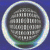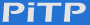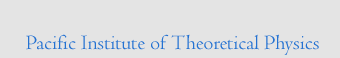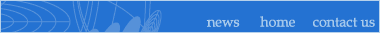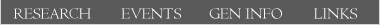Upcoming and Archived: Conferences | Lectures on Quantum Phenomena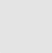## Efficient quantum algorithm for simulating Hamiltonian evolution

### Barry Sanders (University of Calgary)

Date: Jan 24, 2007
Time: 12:30 pm
Location: Hennings 318

There are three main applications of quantum computer algorithms: the hidden subgroup problem (with Shor's factorization algorithm one important example), search problems (e.g. Grover's algorithm), and our interest here: simulation (Feynman's original motivation for the quantum computer as a tool for efficient simulation of quantum evolution as opposed to classical computation, which may be exponentially expensive). We develop an efficient quantum algorithm for simulating quantum evolution for any (unknown) Hamiltonian over any time t. Specifically, when the Hamiltonian acts on n qubits, has at most a constant number of nonzero entries in each row and column, and its norm is bounded by a constant, the cost of the simulation is O(log*n t^{1+1/2k}) for k any integer. In other words the cost of the simulation is nearly constant in n and arbitrarily close to linear in the time of evolution; moreover we prove that an algorithm for simulating general evolution of quantum states cannot be sublinear in t. Our results provide a firm algorithmic foundation for studying a quantum computer, especially with respect to assessing and optimizing the cost of the simulation. In addition our methods may lead to improved simulations for quantum control on classical computers.Research Teams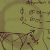Upcoming Events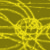General Information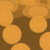Links & Resources